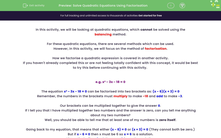# Solve Quadratic Equations Using Factorisation

In this worksheet, students will learn how to solve a quadratic equation of the form x² + bx + c = 0 by finding common factors and factorising.Key stage:  KS 4

Year:  GCSE

GCSE Subjects:   Maths

GCSE Boards:   Pearson Edexcel, OCR, Eduqas, AQA,

Curriculum topic:   Algebra

Curriculum subtopic:   Solving Equations and Inequalities Algebraic Equations

Popular topics:   Factors worksheets

Difficulty level:#### Worksheet Overview

In this activity, we will be looking at quadratic equations, which cannot be solved using the balancing method.

For these quadratic equations, there are several methods which can be used.

However, in this activity, we will focus on the method of factorisation.

How we factorise a quadratic expression is covered in another activity.

If you haven't already completed this or are not feeling totally confident with this concept, it would be best to try this before continuing with this activity.

e.g. x² - 3x - 18 = 0

The equation x² - 3x - 18 = 0 can be factorised into two brackets as: (x - 6)(x + 3) = 0

Remember, the numbers in the brackets must multiply to make -18 and add to make -3

Our brackets can be multiplied together to give the answer 0.

If I tell you that I have multiplied together two numbers and the answer is zero, can you tell me anything about my two numbers?

Well, you should be able to tell me that at least one of my numbers is zero itself.

Going back to my equation, that means that either (x - 6) = 0 or (x + 3) = 0 (They cannot both be zero.)

But if x - 6 = 0 then x must be 6 so x = 6 is a solution.

Alternatively, if x + 3 = 0 then x = -3 is another solution.

So there are two possible solutions to this quadratic equation: x = 6 and x = -3

It is a feature of quadratic equations that they nearly always have two solutions.

Here's another example, this time we'll just include the lines of working you would need to show:

e.g. n² - 10n + 25 = 0

(n - 5)(n - 5) = 0

Either n - 5 = 0 or n - 5 = 0

So n = 5 or n = 5

Now in this case, because both sets of brackets are the same, both solutions are the same, so really there is just one solution: n = 5

This is unusual though; usually there will be two different sets of brackets with two different solutions.

Here's one more example, again slightly different:

e.g. 2y² - 4y = 0

This equation will not factorise into two brackets, but we can take out a common factor of 2y from each term on the left:

2y(y - 2) = 0

Either 2y = 0 or y - 2 = 0

So y = 0 or y = 2

Ok, now you should be ready to try some questions.

In this activity, we will solve quadratic equations in the form x² + bx + c = 0 by finding common factors and factorising into two brackets.

### What is EdPlace?

We're your National Curriculum aligned online education content provider helping each child succeed in English, maths and science from year 1 to GCSE. With an EdPlace account you’ll be able to track and measure progress, helping each child achieve their best. We build confidence and attainment by personalising each child’s learning at a level that suits them.

Get started